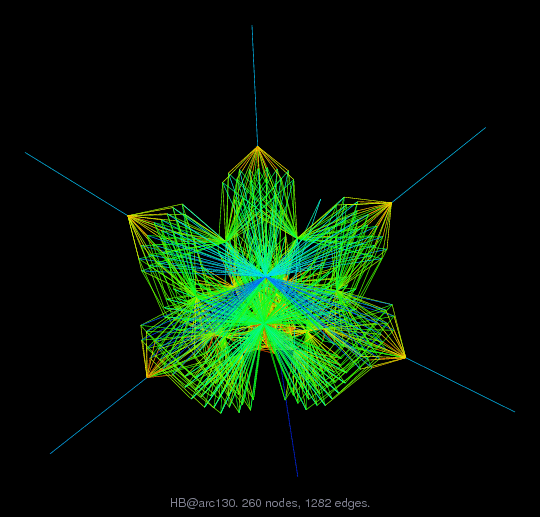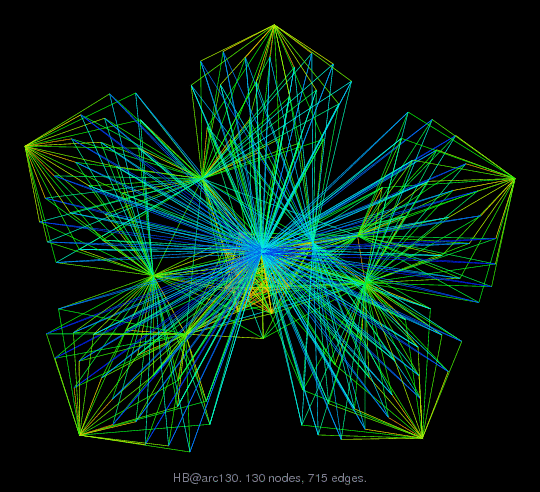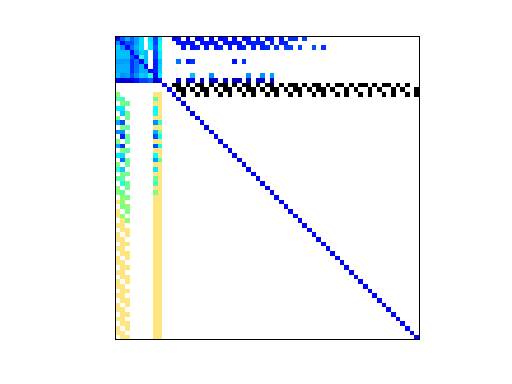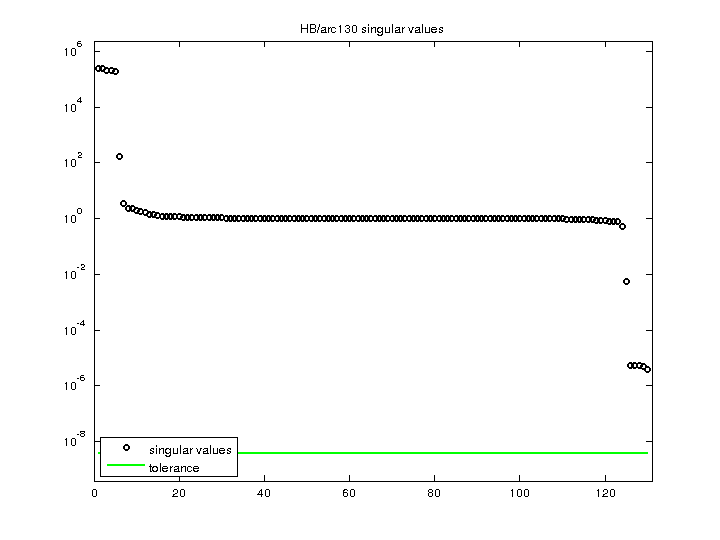Matrix: HB/arc130

Description: UNSYMMETRIC MATRIX FROM LASER PROBLEM. A.R.CURTIS, OCT 1974(bipartite graph drawing) (graph drawing of A+A')• Matrix group: HB
• download as a MATLAB mat-file, file size: 7 KB. Use UFget(6) or UFget('HB/arc130') in MATLAB.

 Matrix properties number of rows 130 number of columns 130 nonzeros 1,037 structural full rank? yes structural rank 130 # of blocks from dmperm 55 # strongly connected comp. 55 explicit zero entries 245 nonzero pattern symmetry 76% numeric value symmetry 0% type real structure unsymmetric Cholesky candidate? no positive definite? no

 author A. Curtis editor A. Curtis, I. Duff, J. Reid date 1974 kind materials problem 2D/3D problem? yes

 Ordering statistics: result nnz(chol(P*(A+A'+s*I)*P')) with AMD 841 Cholesky flop count 6.1e+03 nnz(L+U), no partial pivoting, with AMD 1,552 nnz(V) for QR, upper bound nnz(L) for LU, with COLAMD 982 nnz(R) for QR, upper bound nnz(U) for LU, with COLAMD 2,935

Note that all matrix statistics (except nonzero pattern symmetry) exclude the 245 explicit zero entries.

 SVD-based statistics: norm(A) 239735 min(svd(A)) 3.9598e-06 cond(A) 6.05421e+10 rank(A) 130 sprank(A)-rank(A) 0 null space dimension 0 full numerical rank? yes

 singular values (MAT file): click here SVD method used: s = svd (full (A)) ; status: ok